# Sample size & statistical power in a multiple regression tutorial

2017-10-20

This tutorial will help you compute sample size and statistical power for a multiple regression in Excel using the XLSTAT statistical software.

XLSTAT-Modelling data offers a tool to apply a linear regression model. XLSTAT estimates the power or calculates the necessary number of observations associated with variations of R ² in the framework of a linear regression.

When testing a hypothesis using a statistical test, there are several decisions to take:

• The null hypothesis H0 and the alternative hypothesis Ha.
• The statistical test to use.
• The type I error also known as alpha. It occurs when one rejects the null hypothesis when it is true. It is set a priori for each test and is 5%.

The type II error or beta is less studied but is of great importance. In fact, it represents the probability that one does not reject the null hypothesis when it is false. We can not fix it upfront, but based on other parameters of the model we can try to minimize it. The power of a test is calculated as 1-beta and represents the probability that we reject the null hypothesis when it is false. We therefore wish to maximize the power of the test. The XLSTAT module calculates the power (and beta) when other parameters are known. For a given power, it also allows to calculate the sample size that is necessary to reach that power.

The statistical power calculations are usually done before the experiment is conducted. The main application of power calculations is to estimate the number of observations necessary to properly conduct an experiment.

In a future study, we wish to study the weights of children according to size and age of children (as in the following tutorial on Multiple Linear Regression).

We want to know if the R² of this model is significantly different from 0. There will be two independent variables or predictors and we would like to know how many children should be interviewed to obtain a power of 0.9. Since we do not yet know the parameters of our samples, we will use the concept of effect size. Cohen (1988) introduced this concept which provides an order of magnitude for the effect size. So we will test three effect sizes: 0.02 for a small effect, 0.15 for a moderate effect and 0.35 for a strong effect. It is expected that the larger the effect size is, the smaller the sample size required will be.

## Setting up of the calculation of the required sample size or statistical power in a multiple regression

After opening XLSTAT, click the Power icon and choose linear regression.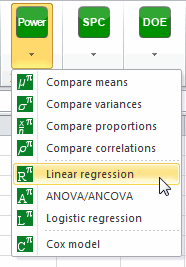Once the button is clicked, the dialog box appears. You must then choose the objective Find the sample size.

Then select the test R² different from 0. The alpha is 0.05.

The desired power is 0.9.

The number of predictors or explanatory variables is 2. Rather than detailed input parameters, we select the effect size option and enter the value 0.02 for a weak effect.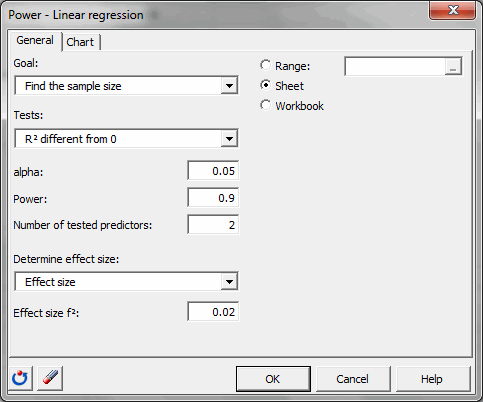In the Charts tab, the option simulation plot is activated and the "size of sample 1" will be displayed on the vertical axis and the "power" on the horizontal axis.

Power varies between 0.8 and 0.95 by increments of 0.01.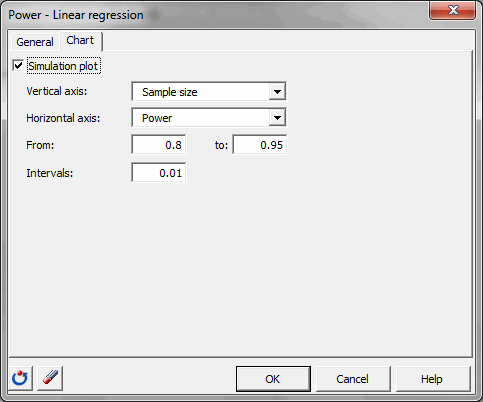Once you have clicked the OK button, the calculations are made, and then the results are displayed.

## Resulst of the calculations of the required sample size or statistical power in a multiple regression

The first table shows the parameters used as input. In our case, only the number of predictors is displayed.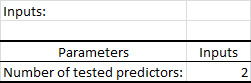The second table shows the calculation results and an interpretation of the results.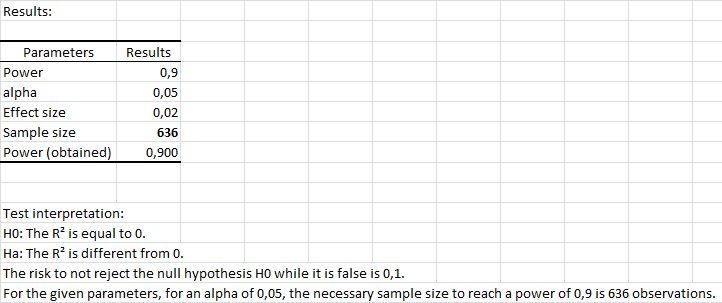We see it takes 636 observations to obtain a power as close as possible to 0.9.

The following table summarizes the calculations obtained for each value of power between 0.8 and 0.95.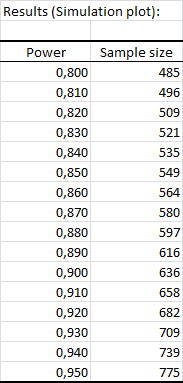The simulation plot shows the evolution of the sample size depending on the power. We see that for a power of 0.8, just a little more than 485 observations and that a power for a power of 0.95, 775 observations are needed.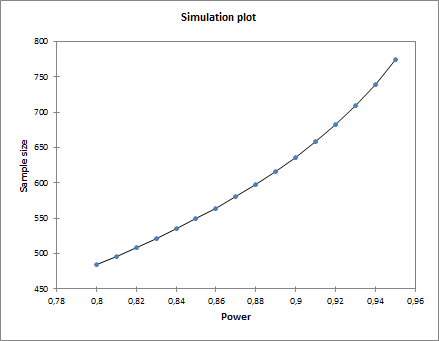For effect sizes of 0.15 and 0.35, we obtain the following results: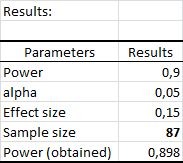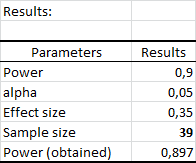The sample size will therefore fall as the R² moves away from 0 and we see that for a large difference, 39 observations will be sufficient.

So if we assume that the quality of explanation of the variables age and weight is strong (R ² close to 1) on the size of a child, 39 observations will be sufficient to obtain a power of 0.9.

XLSTAT is a powerful tool both to investigate the sample size required for an analysis and to calculate the power of a test. Obviously, if the user has more information about the samples or populations, he may give details of the input parameters, rather than using the effect size.

#### Contact our technical support team: support@xlstat.com

https://cdn.desk.com/
false
desk